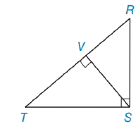Chapter 5.4, Problem 5E### Elementary Geometry for College St...

6th Edition
Daniel C. Alexander + 1 other
ISBN: 9781285195698

#### Solutions

Chapter
Section### Elementary Geometry for College St...

6th Edition
Daniel C. Alexander + 1 other
ISBN: 9781285195698
Textbook Problem
17 views

# Use theorem 5.4.2 to find RV if S V = 6     and V T = 8 .Exercises 1-6

To determine

To Find:

The length of RV.

Explanation

Given SV=6 and VT= 8

And figure,

From the figure,

ΔRST is a right triangle with right angle RST, SV¯RT¯

From the theorem,

The length of the altitude to the hypotenuse of a right triangle is the geometric mean of the lengths of the segments of the hypotenuse.

Since ΔRVSΔRST

Corresponding sides of similar triangles are proportional (CSSTP).

So, we have

VTSV=SVRV

Recall the Means-Extremes Property

In a proportion, the product of the means equals the product of the extremes. That is,

### Still sussing out bartleby?

Check out a sample textbook solution.

See a sample solution

#### The Solution to Your Study Problems

Bartleby provides explanations to thousands of textbook problems written by our experts, many with advanced degrees!

Get Started

#### Evaluate 1(x41+x6)2dx.

Calculus (MindTap Course List)

#### Differentiate. g(x)=1+2x34x

Single Variable Calculus: Early Transcendentals

#### Polar coordinates of the point with rectangular coordinates (5, 5) are: (25, 0)

Study Guide for Stewart's Single Variable Calculus: Early Transcendentals, 8th